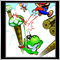# Trying to find last two digits of a number30

I'm trying to find the last two digits of a number.  In this case 1.11858.  I find I first have to multiply the number by 100000 to convert the number to a whole number.  Next I'm trying to find the last two digits by creating a loop, in which the whole number is subtracted by 100 until all that's left is a number which is less than 100.  this number will be the last two digits.  I've tried using the do while loop, but not sure if I'm utilizing it right.  I keep ending up with an endless loop.  Could someone look at this and see if I've missed something or maybe not using it correctly.

Thanks!

```   int l = 0;

M=(1.11858*100000);

do{
N=(M-100);
l++;
}

while(N>100);188

Yellowbeard1781:

I'm trying to find the last two digits of a number.  In this case 1.11858.  I find I first have to multiply the number by 100000 to convert the number to a whole number.  Next I'm trying to find the last two digits by creating a loop, in which the whole number is subtracted by 100 until all that's left is a number which is less than 100.  this number will be the last two digits.  I've tried using the do while loop, but not sure if I'm utilizing it right.  I keep ending up with an endless loop.  Could someone look at this and see if I've missed something or maybe not using it correctly.

Thanks!

A way I've done this before is to subtract the CEILING figure, so multiple 1.11858 * 100000 = 111858

and then round/ceiling it to: 1.118 and x 100000 = 111800

Then it's just the difference 111858 - 111800 = 58188

iRick:

A way I've done this before is to subtract the CEILING figure, so multiple 1.11858 * 100000 = 111858

and then round/ceiling it to: 1.118 and x 100000 = 111800

Then it's just the difference 111858 - 111800 = 58

Sorry mean Floor rather than Ceiling1156

Maybe this works:

```int number=(int)(value*MathPow(10,_Digits)); // 1.01234 -> 101234
int last2digits=number%100; // 101234 -> 34
```
Note that (int) always rounds down, that is 1.99 gets 1. Right code depends on what you're looking for.